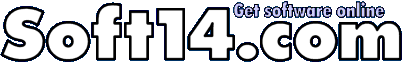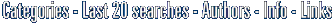(sometimes we provide two download links because it may happen that the main server / link is overloaded or slow or non responding - the files are identical. Just choose one, save to the Desktop, double click to install)

Purchase link: Purchase / Get it now!

## This calculator calculates definite integrals of large variety of functions by tanh-sinh quadrature scheme. Numerical values are calculated with precision 90 digits. Also uncertainty of result is computed. All calculation results are reflected in History rich-text-box, which can be saved in file or printed.

(This calculator calculates definite integrals of large variety of functions.)This calculator computes definite integrals by tinh-sinh quadrature scheme. Type formula into Integrand text-box. Change default values of lower and upper limits of integration. Click Calculate button. Watch if the algorithm is converging. The Current Uncertainty value should be steadily decreasing. If the algorithm is diverging, click Stop button. Observe results of calculation in History tab. At each level the number of calculation points doubles and so does the number of accurate digits. You can watch it by decreasing exponent of current uncertainty. The number of points of calculation depends on level and length of interval of integration for tinh-sinh quadrature algorithm. If you use in integrand functions with accuracy lower than the default for the calculator, then adjust Desired Uncertainty value. The convergence of algorithm is not guaranteed for all functions and all intervals of integration. Tanf-sinh quadrature scheme is fast and and gives right answer for large variety of functions. But for some functions and intervals the algorithm diverges. And for few functions the scheme gives wrong answer.